# AP Chemistry : Thermodynamics

## Example Questions

### Example Question #11 : Thermodynamics

Which of these conditions will result in a spontaneous reaction?

Exothermic reactions with reactants going from gaseous to liquid state

Exothermic reactions with reactants going from liquid to gaseous state

Endothermic reactions with reactants going from gaseous to liquid state

Endothermic reactions with reactants going from liquid to gaseous state

All exothermic reactions

Exothermic reactions with reactants going from liquid to gaseous state

Explanation:

To determine whether a reaction will occur spontaneously, one needs to look at whether the Gibbs free energy will decrease. In order for Gibbs free energy to decrease, the combination of the heat of the reaction and the change in entropy must give a negative Gibbs free energy. The equation is: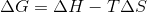If a reaction is exothermic, the heat of the reaction is negative. If the reactants go from liquid to gaseous state, the entropy increases (is positive). When the enthalpy is negative and the entropy is positive, the equation must always give a negative solution. This leads to a negative Gibbs free energy, so the reaction will be spontaneous.

### Example Question #12 : Thermodynamics

Which of the following reaction conditions cannot result in a spontaneous reaction?

Exothermic reactions in which reactants go from liquid to gaseous state

Endothermic reactions in which reactants go from gaseous to liquid state

Endothermic reactions in which reactants go from liquid to gaseous state

Exothermic reactions in which reactants go from gaseous to liquid state

Exothermic reactions in which reactants go from liquid to solid state

Endothermic reactions in which reactants go from gaseous to liquid state

Explanation:

To determine whether a reaction will occur spontaneously, one needs to look at whether the Gibbs free energy will decrease. In order for Gibbs free energy to decrease, the combination of the heat of the reaction and the change in entropy must give a negative Gibbs free energy. The equation is:If a reaction is endothermic, the heat of the reaction is positive. If the reactants go from gaseous to liquid state, the entropy decreases (is negative). When the enthalpy is positive and the entropy is negative, the equation must always give a positive solution. This leads to a positive Gibbs free energy, so the reaction can never be spontaneous.

### Example Question #1 : Endergonic And Exergonic Reactions

Which of the following values must be negative in order for a reaction to be spontaneous?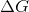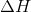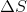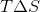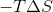Explanation:

In order to determine if a reaction is spontaneous, one needs to look at, which is the change in Gibbs free energy. The formula to find change in Gibbs free energy is:andcan have positive or negative values (under certain conditions), butmust always be negative in order for the reaction to be spontaneous.

### Example Question #1 : Endothermic And Exothermic Reactions

What type of energy conversion occurs during an endothermic reaction?

Kinetic energy to chemical energy

Chemical energy to kinetic energy

Nuclear energy to chemical energy

Kinetic energy to potential energy

Temperature to chemical energy

Kinetic energy to chemical energy

Explanation:

In an endothermic reaction, heat is absorbed and converted into chemical energy. Heat is the sum of molecular kinetic energy in a sample, so kinetic energy is converted into chemical energy. Note that temperature is a measure of heat, and is not a type of energy in itself.

### Example Question #2 : Endothermic And Exothermic Reactions

What will happen to the rate of an exothermic reaction if the temperature is increased?

It will be unaffected

It will increase

It will decrease

It depends on the initial concentrations of the reactants

It will increase

Explanation:

The rate of a reaction will always increase with temperature.

Do not confuse the rate at which a reaction proceeds with the final equilibrium of the reaction. Yes, increasing the temperature of an exothermic reaction will push the equilibrium towards the reactant's side, but this equilibrium will be achieved much more quickly at higher temperatures. Kinetics and thermodynamics are two completely different ways to observe a reaction.

### Example Question #3 : Endothermic And Exothermic Reactions

If a reaction is endothermic and occurs spontaneously, which of the following is not true?

ΔH > 0

ΔS > 0

K = 0

ΔG < 0

K = 0

Explanation:

ΔH is always positive for an endothermic reaction, and ΔG is always negative for a spontaneous reaction. Given the equation delta G = ΔH – T(ΔS), T(ΔS) is positive, so ΔS is positive. We do not know anything about the equilibrium of the reaction.

### Example Question #4 : Endothermic And Exothermic Reactions

If a reaction has a negative entropy and a negative enthalpy value, which of the following terms describes the energy of this reaction?

Exothermic
Exergonic
Endergonic
Endothermic
Constant
Explanation:

Endergonic and exergonic are terms used to describe the free energy of a reaction, so they do not apply in this case since there is not enough information to determine the free energy produced by this reaction without knowing the temperature (delta G = delta H - T delta S). The negative enthalpy allows us to definitively say that the reaction is exothermic.

### Example Question #1 : Endothermic And Exothermic Reactions

Consider the following reversible, exothermic reaction: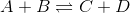The temperature of the vessel in which the reaction is taking place is increased from 300K to 350K. This leads the rate of the reverse reaction to __________.

The change cannot be determined

decrease

increase

stay the same

increase

Explanation:

In exothermic and endothermic reactions, heat can be considered to be either a product or reactant, respectively. Increasing the temperature of the an exothermic reaction is effectively the same as adding heat as a product, causing the reaction to shift to the left. This will cause the reverse reaction to increase in rate.

### Example Question #6 : Endothermic And Exothermic Reactions

If the decomposition of 2 moles of gaseous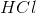into 1 mole each of gaseous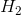and gaseous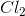at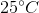absorbs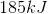of heat energy, then what is the standard heat of formation ofin kilojoules per mole?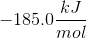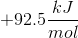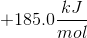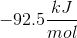Explanation:

From the question stem, we are told that the breakdown of 2 moles of gaseousrequires an absorption ofof energy. For simplification, we can write out the reaction as: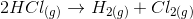By definition, the heat of formation of a compound is the enthalpy change that occurs for the formation (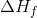) of that compound from its elements in their standard state. Sinceandare in their standard state, we know that they have a heat of formation of zero, thus simplifying the calculation. Thus, if we reverse the above reaction, we see that: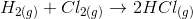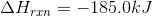Notice that by reversing the reaction, we are also reversing the sign for the enthalpy of the reaction. Now, to calculate theofas kilojoules per mole, we need to multiply the reaction by one-half to obtain: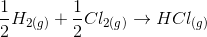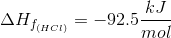### Example Question #7 : Endothermic And Exothermic Reactions

The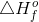of individual products and reactants can be utilized to determine if the reaction is exothermic or endothermic.

Consider the following equation: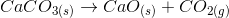Use a standard enthalpy of formation table to calculate the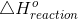, and determine if the reaction is endothermic or exothermic.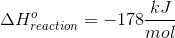, the reaction is exothermic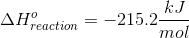, the reaction is endothermic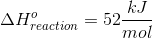, the reaction is exothermic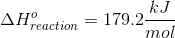, the reaction is exothermic, the reaction is endothermic, the reaction is endothermic

Explanation:

We can calculate the standard change in enthalpy for the reaction using the following equation: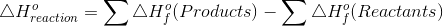of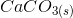of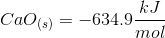of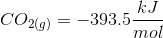Plug in known values and solve.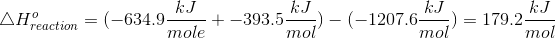A negativeindicates that the reaction is exothermic. This means that the reaction will release energy (usually in the form of heat) to the surroundings.

A positiveindicates that the reaction is endothermic. This means that the reaction will absorb energy (usually in the form of heat) from the surroundings.

Therefore, this overall reaction is endothermic.